Equation of a Straight LineCustom SearchEQUATION OF A STRAIGHT LINE In Mathematics, Volume 1, equations such as 2x+y=6 are designated as linear equations, and their graphs are shown to be straight lines. The purpose of this discussion is to study the relationship of slope to the equation of a straight line. POINT-SLOPE FORM Suppose that we want to find the equation of a straight line that passes through a known point and has a known slope. Let (x,y) represent the coordinates of any point on the line, and let (x,,y,) represent the coordinates of the known point. The slope is represented by m. Recalling the formula defining slope in terms of the coordinates of two points, we haveEXAMPLE: Find the equation of a line passing through the point (2,3) and having a slope of 3. SOLUTION: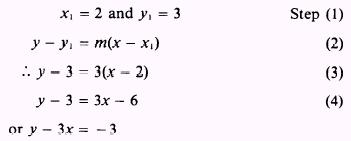The point-slope form may be used to find the equation of a line through two known points. The values of x,, x2, y,, and Y2 are first used to find the slope of the line; then either known point is used with the slope in the point-slope form. EXAMPLE: Find the equation of the line through the points ( - 3,4) and (4, - 2). SOLUTION: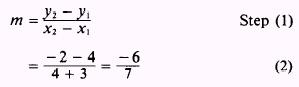Letting (x,y) represent any point on the line and using ( - 3,4) as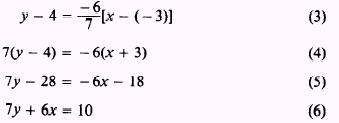Using (4, - 2) as the known point will also give 7y + 6x = 10 as the linear equation. SLOPE-INTERCEPT FORM Any line that is not parallel to the Y axis intersects the Y axis at some point. The x coordinate of the point of intersection is 0, because the Y axis is vertical and passes through the origin. Let the y coordinate of the point of intersection be represented by b. Then the point of intersection is (O,b), as shown in figure 1-8. The y coordinate, b, is called the y intercept. The slope of the line in figure 1-8 isThe value of Ay in this expression is y - b, where y represents the y coordinate of any point on the line. The value of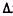x is x - 0 = x, so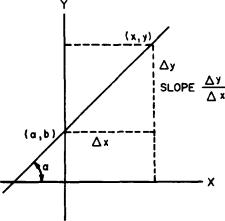Figure 1-8.-Slope-intercept form. This is the standard slope-intercept form of a straight line. EXAMPLE: Find the equation of a line that intersects the Y axis at the point (0,3) and has a slope of 513. SOLUTION:PRACTICE PROBLEMS: Write equations for lines having points and slopes as follows: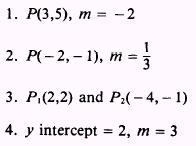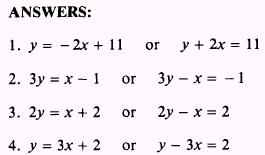NORMAL FORM Methods for determining the equation of a line usually depend upon some knowledge of a point or points on the line. Let's now consider a method that does not require advance knowledge conŁcerning any of the line's points. All that is known about the line is its perpendicular distance from the origin and the angle between the perpendicular and the X axis, where the angle is measured counterclockwise from the positive side of the X axis. In figure 1-9, line AB is a distance p away from the origin, and line OM forms an angle 9 (the Greek letter theta) with the X axis. We select any point P(x,y) on line AB and develop theFigure 1-9.-Normal form. equation of line AB in terms of the x and y of P. Since P represents ANY point on the line, the x and y of the equation will represent EVERY point on the line and therefore will represent the line itself. PR is constructed perpendicular to OB at point R. NR is drawn parallel to AB, and PN is parallel to OB. PS is perpendicular to NR and to AB. A right angle is formed by angles NRO and PRN. Triangles ONR and OMB are similar right triangles. Therefore, angles NRO and MBO are equal and are designated as 8'. Since 8 + 8' = 90' in triangle OMB and angle NRO is equal to 8', then angle PRN equals 8. Finally, the x distance of point P is equal to OR, and the y distance of P is equal to PR. To relate the distance p to x and y, we reason as follows: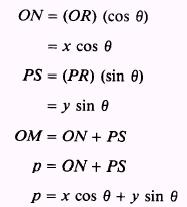This final equation is the normal form. The word "normal" in this usage refers to the perpendicular relationship between OM and AB. "Normal" frequently means "perpendicular" in mathematical and scientific usage. The distance p is always conŁsidered to be positive, and 8 is any angle between 0' and 360'. EXAMPLE: Find the equation of the line that is 5 units away from the origin, if the perpendicular from the line to the origin forms an angle of 30' from the positive side of the X axis. SOLUTION.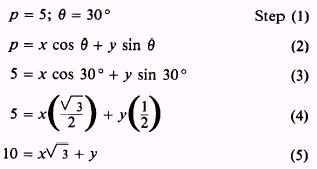Integrated Publishing, Inc. - A (SDVOSB) Service Disabled Veteran Owned Small Business